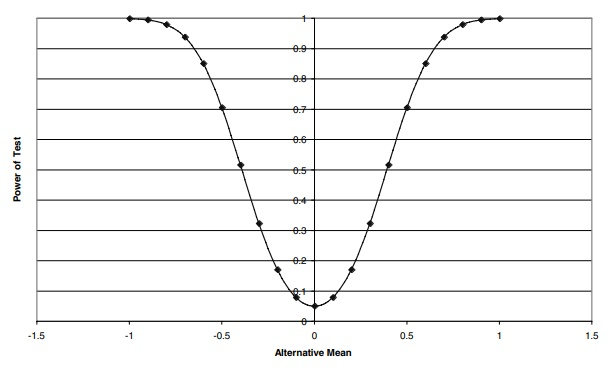# The Power Function

| Home | | Advanced Mathematics |

## Chapter: Biostatistics for the Health Sciences: Tests of Hypotheses

The power function depends on the significance level of a test and the sampling distribution of the test statistic under the alternative values of the population parameters.

THE POWER FUNCTION

The power function depends on the significance level of a test and the sampling distribution of the test statistic under the alternative values of the population parameters. For example, when a Z or t statistic is used to test the hypothesis (H0) that the population mean μ equals μ0, the power function equals α at μ1 = μ0 and increases as moves away from  μ0. The power function approaches 1 as μ1 gets very far from μ0. Figure 9.1 shows a plot of the power function for a population mean in the simple case when μ0 = 0 and is known, the sample size n = 25, and the population distribution is assumed to be a normal distribution.Figure 9.1. Power function for a test that a normal population has mean zero versus a two-sided alterna-tive when the sample size n = 25 and the significance level α = 0.05.

In this case, Z = (– μ1)/ ( σ/√n) = (– μ1)/( σ/5) = 5(– μ1)/σ and Z has a standard normal distribution. This distribution depends on μ1 and σ. We know the value of σ and can take σ = 1, recognizing that although the power depends on μ1 for the curve in Figure 9.1, to be more general we would replace μ1 with μ1/σ for other values of σ. The power is the probability of observing Z in the acceptance region that is P(–C < Z < C), where C is the critical value; consequently, the power depends on the sample size and signif-icance level through C as well as the sample size n through the formula for Z.

Figure 9.2 displays, on the same graph used for n = 25, the comparable results for a sample size n = 100. We see how the power function changes with increased sample size.